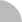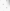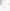# 臺灣博碩士論文加值系統

(34.204.172.188) 您好！臺灣時間：2023/09/27 20:26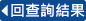:::

### 詳目顯示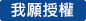: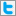Twitter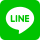•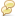被引用:0
•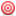點閱:144
•評分: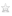•下載:0
•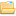書目收藏:0
 本研究是探討在不同的操作參數下沉浸式薄膜過濾系統其過濾系統之表現。 其中操作參數包括溶質種類、濃度和組成，薄膜模組之傾斜角，薄膜種類，曝氣量，透膜壓差，與間歇式操作的運用。在固定壓差法下，利用實驗中各參數的變化來獲得濾速對時間的變化。實驗結果顯示：各實驗系統在曝氣為零的情況下濾速衰退的情況非常快且容易達到與壓差無關的臨界濾速，而曝氣不為零的情況下，在溶質為Dextran T500的系統中，在100k MWCO薄膜中少量曝氣即可以達到與大量曝氣同樣減緩濾速快速衰退的情形，而在500k MWCO 薄膜中不同的曝氣量有著較顯著的不同之濾速提升情形。溶質為PMMA顆粒的系統下，在100k MWCO薄膜中曝氣量的多寡對濾速影響並不明顯且其濾速值與純水濾速相近，而在500k MWCO薄膜中濾速有著比在Dextran T500溶質系統中曝氣有著更明顯的影響。在Dextran T500與PMMA顆粒混合溶液中，溶質比例的不論如何變化，即使在提升大顆粒的附著性系統中(PMMA顆粒濃度高達7.5 kg/m3)，其濾速行為仍然與只有Dextran T500溶質系統的濾速行為相近。薄膜傾斜角度的變化(900-1600) 對於在500k MWCO 薄膜下PMMA溶質系統中濾速的改善非常顯著，而在Dextran T500溶質系統中，在1600下少量曝氣即具有在900下大量曝氣同樣的效果。
 In the study, the performance of the submerged membrane filtration system was investigated in different experimental parameter. and fixed trans-membrane pressure difference method was used. The operated condition in the study included the concentration and composition of the solute, the inclination angle of the membrane module, the rejection rate (MWCO) of membrane, aeration rate, and trans-membrane pressure difference. In the system of no aeration, the flux inclination was very serious and reached the critical flux easily. In the filtration system of Dextran T500, the effect of little aeration rate is equal to the membrane performance of large aeration rate in 100k MWCO membrane, and the different aeration rates had more obvious different effects in 500k MWCO membrane than in 100k MWCO. Dextran T500 was also measured in different aeration rates and inclination angles. The aeration had more effect in the filtration system of PMMA powders than in that of Dextran T500.In the double solute system, Dextran T500 played a major role in the flux behavior of the filtration system even if the concentration of T500 was a quite low concentration. The concentration boundary layer thicknesses calculated by concentration polarization model and boundary layer model were discussed in the study.
 CONTENTSFIGURES IIITABLES IXCHAPTER 1 INTRODUCTION 11.1 PREFACE 11.2 MEMBRANE SEPARATION 11.3 THE DEVELOPMENT AND APPLICATION OF ULTRAFILTRATION MEMBRANE 31.4 OBJECTIVES OF THIS STUDY 9CHAPTER 2 LITERATURE REVIEW 112.1 THE CHARACTERISTICS OF MEMBRANE FILTRATION 112.2 THE CHANGE AND DEVELOPMENT OF WASTEWATER TREATMENT SYSTEM 132.3 A NOVEL WASTEWATER TREATMENT SYSTEM - MEMBRANE BIOREACTOR 152.4 THE OPERATING CHARACTERISTICS OF MBR EQUIPMENT 20CHAPTER 3 THEORETICAL CALCULATION 293.1 THE ANALYSIS OF THE FILTRATION RESISTANCE 293.2 MASS TRANSFER COEFFICIENT AND CONCENTRATION BOUNDARY LAYER 30ACCORDING TO THE BOUNDARY LAYER THEORY, WHEN A FLUID FLOWS THROUGH A PLATE, THE THICKNESS OF THE HYDRAULIC BOUNDARY LAYER IS: 30CHAPTER 4 EXPERIMENTAL APPARATUS AND METHOD 354.1 EXPERIMENTAL APPARATUS 354.2 EXPERIMENTAL METHOD 354.3 MATERIALS 36CHAPTER 5 RESULTS AND DISCUSSION 405.1 THE PURE WATER FLUX OF MEMBRANES 405.2 SINGLE SOLUTE SYSTEM 415.2.1 0.8 μm PMMA system 425.2.2 Dextran T500 system 445.3. BISOLUTE SYSTEM 465.3.1 System with θ=900 465.3.2 System with θ=1600 475.4 EFFECT OF PERIODIC OPERATION 485.5 STUDY OF THE PHENOMENA ON THE MEMBRANE SURFACE 485.6 FILTRATION RESISTANCE 505.7 THEORETICAL FLUX OBTAINED FROM CONCENTRATION BOUNDARY LAYER THICKNESS 53CHAPTER 6 CONCLUSION 100NOMENCLATURE 102REFERENCES 104APPENDIX 110FiguresFigure 1.1 Schematic representations of dead-end filtration and cross-flow filtration 10Figure 2.1 The schematic representation of membrane filtration is by crossflow 25Figure 2.2 Evolution of water reclamation treatment trains 25Figure 2.3Schematic presentation of a bioreactor 26Figure 2.4Under fixed flux method, variation of stabilized flux with TMP for different circulation velocities, T= 20oC, Suspended Solids at 10g/l 26Figure 2.5 Experimental determination of critical flux 27Figure 2.6 Under fixed TMP method, variations of permeate flux with time under step increments of trans-membrane pressure, u = 4 m/s, T= 20oC, Suspended Solids at 10g/l 27Figure 2.7 Trans-membrane pressure changes during long-term constant flux in the membrane bioreactor in stabilized biological conditions 28Figure 4.1 The schematic representation of flat sheet submerged membrane bioreactor 36Figure 4.2 Schematic representation of flat sheet submerged membrane bioreactor 37Figure 5.1 Pure water fluxes at different pressures of new membranes (MWCO=100k & 500k) 56Figure 5.2 SEM photomicrographs of a new 500k membrane at 30KX, 50 KX , 100 KX, 1000 KX were taken from the top surface 56Figure 5.3 SEM photomicrographs of a new 500k membrane at 10 KX, 20 KX, 30KX, 50 KX, 100 KX were taken from the cross section 57Figure 5.4 Calibration curves of gas flow meters no.1 and no.2, respectively. 58Figure 5.5 Fluxes at different trans-membrane pressures and at different aeration amounts (MWCO=100k, θ= 900, C0.8μm PMMA = 3 kg/m3) 58Figure 5.6 Fluxes at different aeration amounts (ΔP=-20 cmHg, MWCO=500k, θ= 900, C0.8μm PMMA = 3 kg/m3) 59Figure 5.7 Fluxes at different aeration amounts (ΔP=-40 cmHg, MWCO=500k, θ= 900, C0.8μm PMMA = 3 kg/m3) 59Figure 5.8 Fluxes at different aeration amounts (ΔP=-60 cmHg, MWCO=500k, θ= 900, C0.8μm PMMA = 3 kg/m3) 60Figure 5.9 Flux behavior in a long period (θ= 900, C0.8μm PMMA = 3 kg/m3, G=0 L/min) 60Figure 5.10 Different retention rates at different trans-membrane pressure differences (θ= 900, C Dextran T500=1.5 kg/m3, G = 0L/min) 61Figure 5.11 Different retention rates at different aeration amounts (ΔP=-30 cmHg, C Dextran T500=1.5 kg/m3, θ= 900) 61Figure 5.12 Fluxes at different trans-membrane pressures (C Dextran T500=1.5 kg/m3, G = 0 L/min, θ= 900) 62Figure 5.13 Fluxes at different aeration amounts (C Dextran T500=1.5 kg/m3, ΔP=-30 cmHg, MWCO=100k, θ= 900) 62Figure 5.14 Fluxes at different aeration amounts (C Dextran T500=1.5 kg/m3, ΔP=-30 cmHg, MWCO=500k, θ= 900) 63Figure 5.15 Fluxes at different aeration amounts (C Dextran T500=1.5 kg/m3, ΔP=-30 cmHg, MWCO=100k & 500k, θ= 900) 63Figure 5.16 Different retention rates at different inclined angles (ΔP=-30 cmHg, MWCO=500k, C Dextran T500=0.75 kg/m3) 64Figure 5.17 Fluxes at different aeration amounts (C Dextran T500 + C0.8μmPMMA =1 kg/m3 + 0.5 kg/m3 =1.5 kg/m3, ΔP=-30 cmHg, MWCO=500k , θ= 900) 64Figure 5.18 Fluxes at different aeration amounts (C Dextran T500 + C0.8μmPMMA =1 kg/m3 + 0.5 kg/m3 =1.5 kg/m3, ΔP=-30 cmHg, MWCO=100k, θ= 900) 65Figure 5.19 Fluxes at different aeration amounts (C Dextran T500 =1.5 kg/m3, ΔP=-30 cmHg, MWCO=100k , θ= 900) 65Figure 5.20 Fluxes at different aeration amounts (C Dextran T500 =1.5 kg/m3, ΔP=-30 cmHg, MWCO=500k , θ= 900) 66Figure 5.21 Fluxes at different aeration amounts (C Dextran T500=0.75 kg/m3 , C0.8μmPMMA= 0.75 kg/m3, ΔP=-30 cmHg, MWCO=100k, θ= 900) 66Figure 5.22 Fluxes at different aeration amounts (C Dextran T500=0.75 kg/m3 , C0.8μmPMMA= 0.75 kg/m3, ΔP=-30 cmHg, MWCO=500k, θ= 900) 67Figure 5.23 Fluxes at different aeration amounts (C Dextran T500=0.5 kg/m3, C0.8μmPMMA= 1 kg/m3, ΔP=-30 cmHg, MWCO=100k, θ= 900) 67Figure 5.24 Fluxes at different aeration amounts (C Dextran T500=0.5 kg/m3, C0.8μmPMMA= 1 kg/m3, ΔP=-30 cmHg, MWCO=500k, θ= 900) 68Figure 5.25 Fluxes at different aeration amounts ( C0.8μmPMMA= 1.5 kg/m3, ΔP=-30 cmHg, θ= 900) is the flux of pure water of 100k MWCO 68Figure 5.26 Fluxes at different aeration amounts ( C0.8μmPMMA= 1.5 kg/m3, ΔP=-30 cmHg, MWCO=500k, θ= 1600) 69Figure 5.27 Fluxes at different aeration amounts (C0.8μmPMMA= 0.75 kg/m3& C Dextran T500=0.75 kg/m3, MWCO=500k, ΔP=-30 cmHg, θ= 1600) 69Figure 5.28 Fluxes at different aeration amounts ( C Dextran T500=0.75 kg/m3, MWCO=500k, ΔP=-30 cmHg, θ= 900) 70Figure 5.29 Fluxes at different aeration amounts ( C Dextran T500=0.75 kg/m3, MWCO=500k, ΔP=-30 cmHg, θ= 1600) 70Figure 5.30 Fluxes at different aeration amounts (MWCO=500k, ΔP=-60 cmHg, θ= 1600) 71Figure 5.31 Fluxes at different aeration amounts (MWCO=500k, ΔP=-60 cmHg, θ= 1600) 71Figure 5.32 Fluxes at different aeration amounts (MWCO=500k, ΔP=-60 cmHg, θ= 1600) 72Figure 5.33 Fluxes at different aeration amounts (MWCO=500k, ΔP=-60 cmHg, θ= 1600) 72Figure 5.34 Fluxes at a periodic suction method (operation: 8 min; pause: 2min) of different aeration amounts (C0.16μmPMMA= 7.5 kg/m3& C Dextran T500=0.75 kg/m3 , MWCO=100k, ΔP= -60 cmHg, θ= 1600) 73Figure 5.35 Fluxes at a periodic suction method (operation: 8 min; pause: 2min) of different aeration amounts (C0.16μmPMMA= 7.5 kg/m3& C Dextran T500=0.75 kg/m3, MWCO=500k, ΔP= -60 cmHg, θ= 1600) 73Figure 5.36 Fluxes at a periodic suction method (operation: 8 min; pause: 2min) of different aeration amounts (C0.16μm PMMA= 7.5 kg/m3& C Dextran T500=0.75 kg/m3, MWCO=100k, ΔP= -60 cmHg, θ= 1600) 74Figure 5.37 Fluxes at a periodic suction method (operation: 8 min; pause: 2 min) of different aeration amounts (C0.16μm MMA= 7.5 kg/m3& C Dextran T500=0.75 kg/m3, MWCO=500k, ΔP= -60 cmHg, θ= 1600) 74Figure 5.38 Size distribution report by intensity of Dextran water solution CDextran T500=0.75 kg/m3 (by TREKINTAL CORP.) 75Figure 5.39 Size distribution report by intensity of PMMA water solution C0.8μmPMMA= 7.5 kg/m3 (by TREKINTAL CORP.) 75Figure 5.40 Size distribution report by intensity of PMMA water solution C0.16μmPMMA= 7.5 kg/m3 (by TREKINTAL CORP.) 76Figure 5.41 SEM photomicrograph of the cake on 500k membrane at 30KX was taken from the top surface (ΔP=-60 cmHg, θ= 1600, C0.16μmPMMA= 7.5 kg/m3, G=4 L/min) 76Figure 5.42 SEM photomicrograph of the cake on 500k membrane at 30KX was taken from the top surface (ΔP=-60 cmHg, θ= 1600, C0.16μmPMMA= 7.5 kg/m3 & C Dextran T500=0.75 kg/m3, G=4 L/min) 77Figure 5.43 SEM photomicrograph of the cake on 500k membrane at 50KX was taken from the top surface (ΔP=-60 cmHg, θ= 1600, C0.16μmPMMA= 7.5 kg/m3, G=4 L/min) 77Figure 5.44 SEM photomicrograph of the cake on 500k membrane at 50KX was taken from the top surface (ΔP=-60 cmHg, θ= 1600, C0.16μmPMMA= 7.5 kg/m3 & C Dextran T500=0.75 kg/m3, G=4 L/min) 78Figure 5.45 SEM photomicrograph of the cake on 500k membrane at 100KX was taken from the top surface (ΔP=-60 cmHg, θ= 1600, C0.16μmPMMA= 7.5 kg/m3, G=4 L/min) 78Figure 5.46 SEM photomicrograph of the cake on 500k membrane at 100KX was taken from the top surface (ΔP=-60 cmHg, θ= 1600, C0.16μmPMMA= 7.5 kg/m3 & C Dextran T500=0.75 kg/m3, G=4 L/min) 79Figure 5.47 SEM photomicrograph of the cake on 500k membrane at 30KX was taken from the cross section (ΔP=-60 cmHg, θ= 1600, C0.16μmPMMA= 7.5 kg/m3, G=4 L/min) 79Figure 5.48 SEM photomicrograph of the cake on 500k membrane at 30KX was taken from the cross section (ΔP=-60 cmHg, θ= 1600, C0.16μmPMMA= 7.5 kg/m3& C Dextran T500=0.75 kg/m3, G=4 L/min) 80Figure 5.49 SEM photomicrograph of the cake on 500k membrane at 50KX was taken from the cross section (ΔP=-60 cmHg, θ= 1600, C0.16μmPMMA= 7.5 kg/m3, G=4 L/min) 80Figure 5.50 SEM photomicrograph of the cake on 500k membrane at 50KX was taken from the cross section (ΔP=-60 cmHg, θ= 1600, C0.16μmPMMA= 7.5 kg/m3& C Dextran T500=0.75 kg/m3, G=4 L/min) 81Figure 5.51 SEM photomicrograph of the cake on 500k membrane at 100KX was taken from the cross section (ΔP=-60 cmHg, θ= 1600, C0.16μmPMMA= 7.5 kg/m3, G=4 L/min) 81Figure 5.52 SEM photomicrograph of the cake on 500k membrane at 100KX was taken from the cross section (ΔP=-60 cmHg, θ= 1600, C0.16μmPMMA= 7.5 kg/m3& C Dextran T500=0.75 kg/m3, G=4 L/min) 82Figure 5.53 SEM photomicrograph of the cake on 500k membrane at 30KX was taken from the top surface (ΔP=-60 cmHg, θ= 1600, C0.16μmPMMA= 7.5 kg/m3, G=0 L/min) 82Figure 5.54 SEM photomicrograph of the cake on 500k membrane at 30KX was taken from the top surface (ΔP=-60 cmHg, θ= 1600, C0.16μmPMMA= 7.5 kg/m3 & C Dextran T500=0.75 kg/m3, G=0 L/min) 83Figure 5.55 SEM photomicrograph of the cake on 500k membrane at 50KX was taken from the top surface (ΔP=-60 cmHg, θ= 1600, C0.16μmPMMA= 7.5 kg/m3, G=0 L/min) 83Figure 5.56 SEM photomicrograph of the cake on 500k membrane at 50KX was taken from the top surface (ΔP=-60 cmHg, θ= 1600, C0.16μmPMMA= 7.5 kg/m3 & C Dextran T500=0.75 kg/m3, G=0 L/min) 84Figure 5.57 SEM photomicrograph of the cake on 500k membrane at 100KX was taken from the top surface (ΔP=-60 cmHg, θ= 1600, C0.16μmPMMA= 7.5 kg/m3, G=0 L/min) 84Figure 5.58 SEM photomicrograph of the cake on 500k membrane at 100KX was taken from the top surface (ΔP=-60 cmHg, θ= 1600, C0.16μmPMMA= 7.5 kg/m3 & C Dextran T500=0.75 kg/m3, G=0 L/min) 85Figure 5.59 SEM photomicrograph of the cake on 500k membrane at 1KX was taken from the cross section (ΔP=-60 cmHg, θ= 1600, C0.16μmPMMA= 7.5 kg/m3, G=0 L/min) 85Figure 5.60 SEM photomicrograph of the cake on 500k membrane at 1KX was taken from the cross section (ΔP=-60 cmHg, θ= 1600, C0.16μmPMMA= 7.5 kg/m3 & C Dextran T500=0.75 kg/m3, G=0 L/min) 86Figure 5.61 SEM photomicrograph of the cake on 500k membrane at 30KX was taken from the cross section (ΔP=-60 cmHg, θ= 1600, C0.16μmPMMA= 7.5 kg/m3, G=0 L/min) 86Figure 5.62 SEM photomicrograph of the cake on 500k membrane at 30KX was taken from the cross section (ΔP=-60 cmHg, θ= 1600, C0.16μmPMMA= 7.5 kg/m3 & C Dextran T500=0.75 kg/m3, G=0 L/min) 87Figure 5.63 SEM photomicrograph of the cake on 500k membrane at 50KX was taken from the cross section (ΔP=-60 cmHg, θ= 1600, C0.16μmPMMA= 7.5 kg/m3, G=0 L/min) 87Figure 5.64 SEM photomicrograph of the cake on 500k membrane at 50KX was taken from the cross section (ΔP=-60 cmHg, θ= 1600, C0.16μmPMMA= 7.5 kg/m3 & C Dextran T500=0.75 kg/m3, G=0 L/min) 88Figure 5.65 SEM photomicrograph of the cake on 500k membrane at 100KX was taken from the cross section (ΔP=-60 cmHg, θ= 1600, C0.16μmPMMA= 7.5 kg/m3, G=0 L/min) 88Figure 5.66 SEM photomicrograph of the cake on 500k membrane at 100KX was taken from the cross section (ΔP=-60 cmHg, θ= 1600, C0.16μmPMMA= 7.5 kg/m3 & C Dextran T500=0.75 kg/m3, G=0 L/min) 89Figure 5.67 Different resistances at different pressure differences (ΔP=-60 cmHg, θ= 900, C0.8μmPMMA= 3 kg/m3) 89Figure 5.68 Different resistances at different pressure differences (ΔP=-30 cmHg, θ= 900, G= 0 L/min) 90Figure 5.69 Different resistances at different inclination angles and aeration rates (MWCO=500k, ΔP=-30 cmHg, C0.8μmPMMA= 1.5 kg/m3) 90Figure 5.70 Different resistances at different solute compositions and aeration rates (MWCO=500k, ΔP=-60 cmHg, θ= 900) 91Figure 5.71 Bubble sizes at the gas flow rate of 1, 2, 3, 4, 5 L/min 92Figure 5.72 Different bubble velocities at different aeration rates (the inclination angle of the module is 900) 93Figure 5.73 The tendency of that boundary layer thickness decreases with the increasing flux (100k MWCO the inclination angle of the module is 900) (C Dextran T500 =1.5 kg/m3, ΔP=-30 cmHg, MWCO=100k , θ= 900) 93Figure 5.74 Different experimental fluxs and theoretical fluxes were at different aeration rates. (μ:Cb, D:Cb) means that the viscosity(μ) and diffusivity(D) are calculated in the calculation of flux all by using the mean concentration (Cb) in the boundary layer; (μ:Ci, D:Cb) means that μ &D are calculated by using the initial feed concentration(Ci) and Cb respectively; (μ:Ci, D:Cm) means that μ &D are calculated by using the Ci and the concentration on the membrane surface (Cm) respectively. (C Dextran T500 =1.5 kg/m3, ΔP=-30 cmHg, MWCO=100k , θ= 900) 94TablesTable 4.1 The list of the flat sheet membrane characteristics 36Table 5.1 Pure water fluxes of new membranes at different trans-membrane pressures 89Table 5.2Size ave and zetapotential of PMMA water solution ( C0.16μm PMMA = 0.75 kg/m3) and DEXTRAN water solution(C Dextran T500 = 0.75 kg/m3) and PMMA& DEXTRAN combined water solution by Malvern Zatamaster ( an instrument measuring size and zetapotential) 89Table 5.3 Experimental parameters in the flux-time experiments. (8;2) means operation: 8min, pause 2min.(0-4) means the aeration is 0 L/min in operation and 4L/min in pause 90Table 5.4 Different Rfp at different membrane MWCOs, aeration amounts and transmembrane pressure differences 91Table 5.5 Different Rfp at different solute species and concentrations, membrane MWCOs, and transmembrane pressure differences 91Table 5.6 Different Rfp at different aeration amounts, and inclined angles 92Table 5.7 Different Rfp at different solute species and concentrations, and aeration amounts 92Table 5.8 Data of estimating the rise velocity of bubble 93Table 5.9 Concentration boundary layer thickness obtained from boundary-layer model 93Table 5.10 Different fluxes obtained from concentration polarization model 93
 Cheryan, M. (1998). Ultrafiltration and Microfiltration Handbook. Pennslvania: Technomic Inc.Côté, P., Buisson, H., Pound, C., & Arakaki, G. (1997). Immersed membrane activated sludge for the reuse of municipal wastewater. Desalination, 113(2-3), 189-196.Defrance, L., & JaffrinMember of Institut Universitaire de France.,M.Y. (1999). Comparison between filtrations at fixed transmembrane pressure and fixed permeate flux: application to a membrane bioreactor used for wastewater treatment. Journal of Membrane Science, 152(2), 203-210.Eikelboom, D. H. (1993). High performance bioreactor, a physiological approach to wastewater treatment with zero sludge production by complete retention. Japan- Netherlands Workshop on Integrated Water Management,Fane, A. G., & Cho, B. D. (2002). Fouling transients in nominally sub-critical flux operation of a membrane bioreactor. Journal of Membrane Science, 209(2), 391-403.Field, R. W., Wu, D., Howell, J. A., & Gupta, B. B. (1995). Critical flux concept for microfiltration fouling. Journal of Membrane Science, 100(3), 259-272.Flemming, H., Schaule, G., Griebe, T., Schmitt, J., & Tamachkiarowa, A. (1997). Biofouling -- the Achilles heel of membrane processes. Desalination, 113(2-3), 215-225.Gander, M., Jefferson, B., & Judd, S. (2000). Aerobic MBRs for domestic wastewater treatment: a review with cost considerations. Separation and Purification Technology, 18(2), 119-130.Gui, P., Huang, X., Chen, Y., & Qian, Y. (2003). Effect of operational parameters on sludge accumulation on membrane surfaces in a submerged membrane bioreactor. Desalination, 151(2), 185-194.Kedem, O., & A. Katchalsky. (1961). A physical interpretation of the phenomenological coefficients of membrane permeaility. The Journal of General Physiology, 45, 143.Krishna, R., Urseanu, M. I., van Baten, J. M., & Ellenberger, J. (1999). Rise velocity of a swarm of large gas bubbles in liquids. Chemical Engineering Science, 54(2), 171-183.Kwon, D. Y., Vigneswaran, S., Fane, A. G., & Aim, R. B. (2000). Experimental determination of critical flux in cross-flow microfiltration. Separation and Purification Technology, 19(3), 169.Li, L. N. (2002). A Study on Gas-Liquid Two-phase Ultrafiltration in Inclined Flat-Plate Membrane Modules. Taipei: Master thesis of Graduate Institute of Chemical Engineering, Tamkang University (in Chinese)Lin, C. T. (2001). Membrane Ultrafiltration for Suspension Solutions of Macromolecules. Taipei: Master thesis of Graduate Institute of Chemical Engineering, Tamkang University (in Chinese)Michaels, A. S. (1968). New separation techique for the chemical process industries. Chemical Engineering Progress, 64, 31-43.Nagaoka, H., Ueda, S., & Miya, A. (1996). Influence of bacterial extracellular polymers on the membrane separation activated sludge process. Water Science and Technology, 34(9), 165-172.Nakao, S., Nomura, T., & Kimura S. (1979). Characteristics of macrocular gel layer formed on ultrafiltration tubular membrane. American Institute of Chemical Engineers Journal, 25, 615-622.Ognier, S., Wisniewski, C., & Grasmick, A. (2004). Membrane bioreactor fouling in sub-critical filtration conditions: a local critical flux concept. Journal of Membrane Science, 229(1-2), 171-177.Pan, S. Y. (2003). A study on the Critical Flux of Submerged Membrane Filtration System. Taipei: Master thesis of Graduate Institute of Chemical Engineering, Tamkang University (in Chinese).Schlichting, H., Gersten, K., Krause, E., & Oertel, H. J. (2000). Boundary-layerTheory. Berlin: Springer.Schweitzer, P. A. (1997). Handbook of Separation Techniques for Chemical Engineers (Third Edition ed.). New York: McGraw-Hill.Shimizu, Y., Okuno, Y., Uryu, K., Ohtsubo, S., & Watanabe, A. (1996). Filtration characteristics of hollow fiber microfiltration membranes used in membrane bioreactor for domestic wastewater treatment. Water Research, 30(10), 2385-2392.Sondhi, R., & Bhave, R. (2001). Role of backpulsing in fouling minimization in crossflow filtration with ceramic membranes. Journal of Membrane Science, 186(1), 41-52.Ueda, T., Hata, K., Kikuoka, Y., & Seino, O. (1997). Effects of aeration on suction pressure in a submerged membrane bioreactor. Water Research, 31(3), 489-494.van Dijk, L., & Roncken, G. C. G. (1997). Membrane bioreactors for wastewater treatment: the state of the art and new developments. Water Science and Technology, 35(10), 35-41.Wijmans, J. G., Nakao, S., van den Berg,J. W. A., Troelstra, F. R., & Smolders, C. A. (1985). Hydrodynamic Resistance of Concentration Polarization Boundary Layers in Ultrafiltration. Journal of Membrane Science, 22, 117.Wintgens, T., Rosen, J., Melin, T., Brepols, C., Drensla, K., & Engelhardt, N. (2003). Modelling of a membrane bioreactor system for municipal wastewater treatment. Journal of Membrane Science, 216(1-2), 55-65.Yeh, H. M., & Cheng, T. W. (1999). Analysis of the slip effect on the permeate flux in membrane ultrafiltration. Journal of Membrane Science, 154(1), 41-51.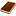國圖紙本論文推文當script無法執行時可按︰推文 網路書籤當script無法執行時可按︰網路書籤 推薦當script無法執行時可按︰推薦 評分當script無法執行時可按︰評分 引用網址當script無法執行時可按︰引用網址 轉寄當script無法執行時可按︰轉寄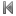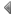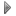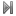top
 1 沉浸式薄膜過濾系統臨界濾速之探討 2 沉浸式薄膜過濾系統中蛋白質結垢之探討

 1 孫明賢。1992。台灣農業的永續發展。中華農學會報（新），160: 1-6。 2 陳仁炫、翁玉娥、王銀波。1994。有機質肥料的添加對土壤磷吸附特性的影響。中國農業化學會誌，32: 332-346。 3 林家棻、李子純、張愛華、陳卿英。1973。長期連用同樣肥料對於土壤化學性質與稻榖收量之影響。農業研究，22: 241-292。 4 王銀波、黃山內、趙震慶。1996。有機農耕法作物養分吸收與殘留之評估。中華農學會報（新），173: 103-119。 5 張正賢、曾美倉。1982。水稻產量構成因素及其影響因子。科學農業，30: 318-322。

 1 藥物溶解速率及邊界層阻力對藥物釋放之影響 2 具固定質量通量和迴流效應的平板式二行程層狀逆流型質量交換器效率改善之研究 3 學校組織信任影響因素之研究 4 網路教學平台視覺化介面影音討論工具之發展 5 應用於軸流式泵之單軸磁力軸承設計與控制改善 6 建構嵌入式車牌辨識系統 7 通貨膨脹目標化與資本累積:一個不完全競爭市場下的總體模型 8 壓電晶片振盪對流場中速度分布之影響 9 台灣產險業之資本經營績效與承接風險資本適足關聯性之研究 10 狹點技術在環保製程熱能整合應用上之研究 11 流動形態對薄膜管中超過濾效能之影響 12 插棒繞線圈型管式超過濾器中改變線圈距離對濾速的影響 13 多孔型聚偏二氟乙烯薄膜固定離胺酸與己二胺 14 離胺酸樹枝狀高分子的製備與其反應機構與物性的研究 15 生物可分解PHA材料包覆四環素藥物之釋放研究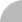簡易查詢 | 進階查詢 | 熱門排行 | 我的研究室